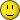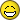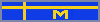Board GamesSubdomains RPGs Video Games Events Login SEARCH Board Game Artists Designers Publishers Accessories Families Forums GeekLists Honors Tags Wiki Users Podcast Podcast Ep. Adv. Search BrowseDatabase User Submitted Content Podcasts ForumsGeekListsBazaareBay MiscAdd to Database HelpThe Hotness
Games|People|Company
 Tsukuyumi: Full Moon Down Die Hard: The Nakatomi Heist Board Game Wingspan Dune Pangea Root Gloomhaven Valley of the Kings: Premium Edition Res Arcana Call to Adventure Terraforming Mars Spirit Island Carnival Zombie Valley of the Kings Carnival Zombie (Second Edition) Architects of the West Kingdom At the Gates of Loyang Scythe The Quacks of Quedlinburg The Lord of the Rings: Journeys in Middle-earth Arkham Horror: The Card Game Underwater Cities Fuji Koro Crusaders: Thy Will Be Done Brass: Birmingham Teotihuacan: City of Gods Gaia Project Paths of Glory Everdell Chronicles of Crime Valley of the Kings: Afterlife The 7th Continent Welcome To... The Silver River Twilight Imperium (Fourth Edition) Great Western Trail UBOOT: The Board Game Azul Fine Sand Age of Steam Rising Sun Nemesis Gold West Root: The Underworld Expansion Kingdom Death: Monster Concordia Middara The Castles of Burgundy That's Pretty Clever Valley of the Kings: Last Rites
12 Posts

## Subject: Probability question for the Mathletes.Your Tags: Add tags Popular Tags: [View All]
I posted a while ago a question about this dice game I am creating. I am back to the drawing board at this point and have some more probability questions surrounding it. Any answers are very appreciated since I do not possess the knowledge nor the technology to solve these.

A d4, d6, d8, d10, and d12 are rolled together. What is the probability that:

1) there is at least one pair

2) there are two pairs

3) there is at least three of a kind (include 4 and 5 of a kind)

4) there is at least four of a kind (include 5 of a kind)

5) there is at least a run of three (include runs of 4 and 5)

6) there is at least a run of four (include 5)

The previous post from over a year ago seemed to agree that there are over 20,000 possible outcomes. And as good as those posters answered my questions, I cannot figure this out on my own.

• [+] Dice rolls
This is definitely something you're going to have to do via simulation rather than an exact proof, although I think that 23,040 combinations is small enough to exhaust rather than doing a Monte-Carlo simulation approach. Just to check, when you look for "at least one pair" and "two pairs", are you including the possibility of 3+-of-a-kinds as you do for the other ones? In particular, does four of a kind include 2 pairs?

I will mock up some R code to run this, and see what I can get.
• [+] Dice rolls
Thanks, everyone, for the responses. I have recently discovered anydice.com, but have yet to master the language of inputs.

Can anyone figure the two pair probability result?

• [+] Dice rolls
Edit: Here's a wrong answer for two pair.Here's an exact answer for two pair (I think):

If the d4 is unpaired:
4 (for the choices on the d4) times 156 = 624
d6/d8 paired: 6*10 =60 (choose the result on the d6 and d10)
d6/d10 paired: 6*8 =48
d6/d12 paired: 6*8 =48

d6 unpaired:

6 times 102 = 612

d4/d8 paired: 4*10 =40
d4/d10 paired: 4*8 = 32
d4/d12 paired: 4*8 = 32

etc:

d8 unpaired:

8 * (40 + 24 + 24) = 704

d10 unpaired:

10*(32 + 24 + 24) = 800

d12 unpaired:

12*(32 + 24 + 24) = 960

Total: 960 + 800 + 704 + 612 + 624 = 3700

And 3700/23040 is about 16.06%.

See? Exact answers can be proved. It's just kind of a pain.• [+] Dice rolls
Joe McKinley
United States
San Jose
California
When I heard the learn’d astronomer; When the proofs, the figures, were ranged in columns before me; When I was shown the charts and the diagrams, to add, divide, and measure them; When I, sitting, heard the astronomer, where he lectured withmuch applause in the lecture-room, How soon, unaccountable, I became tired and sick; Till rising and gliding out, I wander’d off by myself, In the mystical moist night-air, and from time to time, Look’d up in perfect silence at the stars. W.W.
myersd37 wrote:
A d4, d6, d8, d10, and d12 are rolled together. What is the probability that:

1) there is at least one pair

2) there are two pairs

3) there is at least three of a kind (include 4 and 5 of a kind)

4) there is at least four of a kind (include 5 of a kind)

5) there is at least a run of three (include runs of 4 and 5)

6) there is at least a run of four (include 5)

1) there is at least one pair = 16320/23040 = 17/24 ~= 70.83%

2) there are two pairs = 2728/23040 = 341/2880 ~= 11.84%

3) there is at least three of a kind = 2336/23040 = 73/720 ~= 10.14%

4) there is at least four of a kind = 152/23040 = 19/2880 ~= 0.66%

5) there is at least a run of three = 7076/23040 = 1769/5760 ~= 30.71%

6) there is at least a run of four = 1726/23040 = 863/11520 ~= 7.49%

edit: for #2 above, I assumed the 2 pair must be distinct. I.e. a 4 or 5 of a kind does not count as two pair. I also assumed that a full house counts as two pair.
• [+] Dice rolls
I think I could write a program to solve this in anydice if you were rolling a bunch of identical dice, but I'm not sure if there's a way to define a heterogeneous collection of dice (I can't find any in the documentation). I've sent a question to the author.
• [+] Dice rolls
My two pair does count 4 of a kinds and full houses as two pair - that might account for some of the differences.

Edit: And argh, I see. I've double counted full houses. I'll edit the first post.
• [+] Dice rolls
Ok, brute force for the win!

Assumptions: *any* pair allows for things like triples, two pair, etc, two pair allows for full house or quad.

Number of rolls = 23040
Number of "any pair" = 16320
Number of "any 2 pair" = 2880
Number of "any triple" = 2336
Number of "any quad" = 152
Number of "run of 3" = 6134
Number of "run of 4" = 1432

Number of "run of 3" = 7076
Number of "run of 4" = 1726

Probability of:
Pair = 70.83%
Two pair = 12.50%
Triple = 10.14%
Run of 3 = 26.62%
Run of 4 = 6.22%

Run of 3 = 30.71%
Run of 4 = 7.49%

I notice that my results line up with The Unbeliever's for the "of a kinds" except for 2 pair, where the difference is exactly the number of quads. I suspect my run-detection function is poorly written, which would explain why I don't get the same results for runs. I will do a quick bug-check and come back to it.

EDIT: Found the bug. Now to fix it ...

EDIT 2: Fixed, and I get the same results as The Unbeliever.
• [+] Dice rolls
myersd37 wrote:
I posted a while ago a question about this dice game I am creating. I am back to the drawing board at this point and have some more probability questions surrounding it. Any answers are very appreciated since I do not possess the knowledge nor the technology to solve these.

A d4, d6, d8, d10, and d12 are rolled together. What is the probability that:

There are 4*6*8*10*12 possibilities = 23,040

With all the different size dice, calculating probabilities is very tedious, because, at least as far as I know how to do it, you have to consider every combination of different size dice. When the dice are all, say, d6, then all the combinations are the same and you can just multiply by the number of dice or take a combination or some such.

Quote:

1) there is at least one pair

This one's easy.

The easiest way to calculate "at least one" is often to calculate the probability of none and then subtract this from 100%. So let's count the number of ways that no pair of dice are the same.

There are 4 possibilities for the d4. Whatever you roll, the d6 has 5 values different from the d4. Whatever you roll for the d4 and the d6, that leaves 6 possible values for the d8 that match neither the d4 nor the d6. Etc. So the total number of ways that all the dice could be different is:

n=4*5*6*7*8=6720.

So at least one pair is 23,040 - 6,720 = 16,320.

The probability is then 16,320 / 23,040 = 71%

Quote:

2) there are two pairs

You mean exactly 2 or at least 2?

Quote:

3) there is at least three of a kind (include 4 and 5 of a kind)

This is more complicated because you have to be careful not to count the same combination twice. The safe thing to do is to calculate the probabilities of each number, i.e. find exactly 3, exactly 4, and exactly 5, and add together. There may well be an easier way, but here's the first way that comes to my mind.

Again, this is made a lot harder because the dice are all different sizes. You have to deal with many individual cases.

Ways to get exactly 3 of a kind:

d4=d6=d8, d10 & d12 different

4*1*1*9*11=396

d4=d6=d10, not d8 or d12

4*1*1*7*11=308

d4=d6=d12, not d8 or d10

4*1*1*7*9=252

d4=d8=d10, not d6 or d12

4*1*1*5*11=220

d4=d8=d12, not d6 or d10

4*1*1*5*9=180

d4=d10=d12, not d6 or d8

4*1*1*5*7=140

d6=d8=d10, not d4 or d12
Must consider when that number is 1-4 and so could match the d4, versus when it couldn't

4*1*1*3*11=132
2*1*1*4*11=88

d6=d8=d12, not d4 or d10

4*1*1*3*9=108
2*1*1*4*9=72

d6=d10=d12, not d4 or d8

4*1*1*3*7=84
2*1*1*4*7=56

d8=d10=d12, d4 & d6 different
Must consider 1-4 could match either d4 or d6, 5-6 could match d6 but not d4, 7-8 can't match either

4*1*1*3*5=60
2*1*1*4*5=40
2*1*1*4*6=48

Add all those up and we get

396+308+252+220+180+140+132+88+108+72+84+56+60+40+48=2184

Now let's count ways to get exactly 4 of a kind:

d4=d6=d8=d10, not d12

4*1*1*1*11=44

d4=d8=d10=d12, not d6
4*1*1*1*5=20

d4=d6=d10=d12, not d8
4*1*1*1*7=28

d4=d6=d8=d12, not d10
4*1*1*1*9=36

d6=d8=d10=d12, not d4
Two groups again

4*1*1*1*3=12
2*1*1*1*4=8

Total=44+20+28+36+12+8=148

Number of ways to get all 5 the same:

4*1*1*1*1=4

So 3+4+5 of a kind = 2184+148+4=2336

2336 / 23,040 = 10%

Disclaimer: I haven't double-checked the above. I may be missing a combination or have an arithmetic error.

Quote:

4) there is at least four of a kind (include 5 of a kind)

Oh, we've already done the hard part above. Just take the 4 and 5:

148+4=152

152 / 23,040 = 0.7%

Quote:

5) there is at least a run of three (include runs of 4 and 5)

Well, et cetera. As much fun as this is I really should get back to work. :-)

Quote:

6) there is at least a run of four (include 5)

The previous post from over a year ago seemed to agree that there are over 20,000 possible outcomes. And as good as those posters answered my questions, I cannot figure this out on my own.

If you have a lot of these, it might be easier to make a big spreadsheet of all the combinations and write macros to count them. Or if you know anything about programming, write a program.

This is why I generally prefer to stick to dice of the same size: It's much easier to calculate!

Like what's the probability of getting 3 or more the same on 5d8?

n(3)=8*1*1*7*7*5C3=392*10=3920
n(4)=8*1*1*1*7*5C4=56*5=280
n(5)=8*1*1*1*1*5C5=8*1=8
sum=4208

total possibilities = 8^5 = 32,768

p=4208/32768=13%

Update: I saw a mistake in my calculations. Fixed. That cleared up the discrepancy with another poster.
• [+] Dice rolls
 Front Page | Welcome | Contact | Privacy Policy | Terms of Service | Advertise | Support BGG | FeedsGeekdo, BoardGameGeek, the Geekdo logo, and the BoardGameGeek logo are trademarks of BoardGameGeek, LLC.